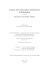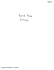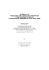# Regular and chaotic regimes in Saltzman model of glacial climate

## Transcription

Regular and chaotic regimes in Saltzman model of glacial climate
```Eur. Phys. J. B (2014) 87: 227
DOI: 10.1140/epjb/e2014-50208-0
THE EUROPEAN
PHYSICAL JOURNAL B
Regular Article
Regular and chaotic regimes in Saltzman model of glacial climate
dynamics under the influence of additive and parametric noise
Dmitry V. Alexandrov1, Irina A. Bashkirtseva1 , Sergei P. Fedotov2 , and Lev B. Ryashko1,a
1
2
Department of Mathematical Physics, Ural Federal University, Lenina ave., 51, 620000 Ekaterinburg, Russia
School of Mathematics, The University of Manchester, Oxford Road, Manchester M13 9PL, UK
Received 26 March 2014 / Received in ﬁnal form 18 April 2014
c EDP Sciences, Società Italiana di Fisica, Springer-Verlag 2014
Published online 7 October 2014 – Abstract. It is well-known that the climate system, due to its nonlinearity, can be sensitive to stochastic
forcing. New types of dynamical regimes caused by the noise-induced transitions are revealed on the basis
of the classical climate model previously developed by Saltzman with co-authors and Nicolis. A complete
parametric classiﬁcation of dynamical regimes of this deterministic model is carried out. On the basis of
this analysis, the inﬂuence of additive and parametric noises is studied. For weak noise, the climate system
is localized nearby deterministic attractors. A mixture of the small and large amplitude oscillations caused
by noise-induced transitions between equilibria and cycle attraction basins arise with increasing the noise
intensity. The portion of large amplitude oscillations is estimated too. The parametric noise introduced
in two system parameters demonstrates quite diﬀerent system dynamics. Namely, the noise introduced in
one system parameter increases its dispersion whereas in the other one leads to the stabilization of the
climatic system near its unstable equilibrium with transitions from order to chaos.
1 Introduction
It is well-known that the variations of the global ice volume of the last million years contain a certain number of
pronounced irregular peaks [1,2]. This behavior responsible for the cyclic character of glaciations has been studied
by many investigators to describe long-term climatic variability of the limit cycle type [3,4]. Such self-oscillations
were shown to appear quite naturally from the coupling
between mean ocean temperature and sea ice extent .
A closure scrutiny of the variance spectra of the global
ice volume shows the presence of random structures superimposed on the irregular peaks. These structures appear
due to aperiodic dynamics in climate systems. The simplest case of periodic forcing leading to the system dynamics with a quasi-periodic attractor was previously studied
in reference . The presence of several variables and parameters and of the external forcing makes it diﬃcult to
disentangle the basic mechanisms responsible for the aperiodic climate dynamics.
In recent years it has been pointed out that the climate system is capable of producing irregular sustained
self-oscillations [7,8]. This autonomous behavior is connected with possibilities for internal and external feedbacks of both positive and negative sign that tend to produce oscillatory, damping or amplifying dynamics of the
earth’s climatic system on many time scales (e.g., Earth
orbital radiation and anthropogenic CO2 changes, changes
a
e-mail: [email protected]
in the atmospheric composition and temperature due to
unpredictable volcanic eruptions, the natural variability of
system variables due to uncertainties in their initial conditions and due to the presence of stochastic noises, etc.). It
should be noted that the quasi-periodic and irregular climatic variations dependent of these feedbacks have been
revealed in a number of geological records [9–12].
A number of simple climatic feedback oscillator models involving sea-ice extent and mean ocean temperature
have been developed earlier by Saltzman et al. [13–16] and
Nicolis [6,17].
All of these models are based on a feedback loop arising from the positive insulating eﬀect of sea ice on temperature, and from the negative eﬀect of temperature on
sea ice. Because it is too diﬃcult to model completely
and accurately the physics involved in the complex feedbacks, this incompleteness is considered as a stochastic
process (noise). Also note that some of the high-frequency
phenomena that act in the climatic system can be represented by stochastic perturbations . The examination
of noise-induced transitions between possible climatic system attractors is the principal goal of the present article.
It is worth noting that due to nonlinearity, noise can
induce new dynamical regimes which have no analogue in
the deterministic case. Noise-induced phenomena in biophysics, electronics, mechanics, population dynamics, geophysics attract an attention of many researchers [19–26].
Potentially, the long-term climatic changes in the form
of aperiodic self-sustained oscillations may arise from the
Page 2 of 10
Eur. Phys. J. B (2014) 87: 227
interaction between surface energy balance and mass balance of the cryosphere. The sea-ice coverage (dependent
on the ocean temperature) can either prevent the loss of
heat from the ocean in high latitudes by its insulating effect, thereby warming the bulk ocean (negative feedback),
or cool the ocean by the ice-albedo eﬀect (positive feedback). In addition, positive feedback is also caused by the
water vapor and CO2 eﬀects on ocean temperature.
A principal model describing the interaction between these processes is the one proposed by Saltzman
et al. [13–16]. This model consists of the following coupled autonomous equations governing the departures of
the sine of latitude of sea ice extent η and of the mean
ocean temperature θ from their equilibrium values
dη = φ1 θ − φ2 η ,
dτ
dθ
= −ψ1 η + ψ2 θ − ψ3 η 2 θ ,
dτ
(1)
where positive constants φ1 , φ2 , ψ1 , ψ2 and ψ3 are detailed
previously by Saltzman et al.
The linear terms in the right-hand sides of system (1)
describe damped harmonic oscillations arising due to the
“ice-insulator” eﬀect (negative feedback) and a destabilizing eﬀect caused by the positive feedback between atmospheric CO2 concentration and mean temperature of the
ocean. A non-linear restorative mechanism that is dominant when the system is far from its equilibrium is described by the term proportional to η 2 θ (the detailed
explanation of this term is given in Ref. ).
Let us introduce the following scaling transformations
√
ψ3 φ1 ψ3 φ1 ψ1
ψ2
x=
η, y=
θ , t = φ2 τ, a =
2 , b = φ
3/2
φ2
φ
2
φ
2
2
according to references [6,17]. In this case, system (1)
becomes
dx
= y − x,
dt
dy
= −ax + by − x2 y.
dt
(2)
Now we see that the deterministic dynamics is entirely
determined by two dimensionless parameters a and b only.
Let us summarize the main features of the aforementioned model previously analyzed by Saltzman with coauthors and Nicolis in a narrow domain of system parameters. For any values of a and b, system (2) has a trivial
equilibrium M0 (0, 0) stable for b < 1 and b < a. The system behavior in this region is quite simple: all trajectories
are attracted to the stable equilibrium M0 . Then, when
the system parameters pass through the line a > 1, b = 1
due to Andronov-Hopf bifurcation, the equilibrium M0
loses its stability so that the stable auto-oscillations are
born. Note that this sustained oscillation regime was used
by Saltzman for theoretical explanation of the global climatic changes. For ﬁxed parameters a = 6.4, b = 4, these
oscillations forced by additive noise were studied in reference  by means of direct numerical simulations. Then,
an analytical investigation of sustained oscillations with
additive noise near Andronov-Hopf bifurcation border has
been developed in reference  with the help of the normal form technique. The eﬀect of a periodic forcing in the
right-hand side of dimensionless system (2) in the vicinity
of the homoclinic bifurcation point a = b = 1 was studied
in reference . Thus, the deterministic model (2) of the
“mean ocean temperature – sea-ice” system was investigated only for severely limited parametric zones in spite
of the fact that possible estimates of system parameters
a and b may lie out of the analyzed interval (see their
diﬀerent estimates given in Refs. [14–16]). In the case of
stochastic dynamics, only the inﬂuence of additive random noise was previously considered in references [15,17].
Therefore, the “mean ocean temperature – sea-ice” model
behavior should be studied more precisely in a broad region of possible values of the system parameters in both
cases of deterministic and stochastic dynamics. Here it is
necessary to investigate the inﬂuence not only additive but
inevitably present parametric noise. This goal explains the
outline of the present study.
First, in Section 2, a full classiﬁcation of all typical
dynamic regimes for the classical two-dimensional deterministic climate model (2) is given via bifurcation analysis. Here, qualitative changes of the phase portraits are
discussed. Note that in previous papers only particular
regimes were studied. This classiﬁcation of deterministic
regimes serves as a basis for understanding the probabilistic mechanisms of the stochastic phenomena.
Second, the inﬂuence of additive noise on these dynamical regimes is studied in Section 3, where diﬀerent
types of noise-induced mixed-mode oscillations are discussed. It is shown how due to noise-induced transitions
between deterministic attractors (equilibria and cycle) a
new stochastic regime with intermittency between smalland large-amplitude stochastic oscillations is formed. For
this model, two new stochastic phenomena are shown: a
generation of large-amplitude stochastic oscillations in a
parametric zone where the deterministic model has stable
equilibria only; a generation of small-amplitude stochastic
oscillations in a parametric zone where the deterministic
model has a stable cycle only.
Furthermore, some unexpected peculiarities of the system response on parametric noise are studied in Section 4.
Note that the inﬂuence of parametric noise on this model
has never been documented previously. In our paper, we
present a new phenomenon of the localization of system
states near the unstable equilibrium due to parametric
noise. An interesting relationship of such phase localization with transition to chaos is ﬁrst established and
discussed.
2 Overview of deterministic dynamics
It is well-known that a mutual arrangement of equilibria
and cycles of the deterministic system (2) plays an important role for the understanding of the variety of its dynamical regimes. Qualitatively diﬀerent types of system dynamics are shown in Figure 1. Let us initially consider the
Eur. Phys. J. B (2014) 87: 227
a = 0.7
Page 3 of 10
a = 0.76
a = 0.86
a = 1.5
Fig. 1. Dynamics of the deterministic model for b = 2: stable equilibria (blue circles), unstable equilibria (red circles), stable
cycles (blue solid curves), unstable cycles (red dotted curves), phase trajectories (black curves).
case b > a, when system (2), along with the trivial equilibrium M0 , has a pair of nontrivial symmetric
√ equilibria
M1 (x̄1 , √
ȳ1 ) and M2 (x̄2 , ȳ2 ). Here x̄1 = ȳ1 = b − a, x̄2 =
ȳ2 = − b − a. In the case b < 1 only two simple diﬀerent types of phase portraits are possible (the case without
cycles): (1) for 0 < a < b, the equilibrium M0 is unstable
and M1 , M2 are stable; (2) for a > b, system (2) has a
single stable equilibrium M0 .
Now consider in detail the case b > 1. Let us ﬁx b = 2
for deﬁniteness and change the second system parameter a. As a result, one can mark four bifurcation values:
a1 ≈ 0.714, a2 ≈ 0.775, a3 = 1, and a4 = 2. These points
deﬁne the following intervals of the structural stability:
A (0 < a < a1 ), B (a1 < a < a2 ), C (a2 < a < a3 ),
D (a3 < a < a4 ), E (a > a4 ). For any zone, system (2)
exhibits an intrinsic type of dynamics.
Dynamics of system (2) in zone A is determined by
the presence of the unstable equilibrium M0 and two stable equilibria M1 , M2 . Here, a phase trajectory tends to
M1 or M2 depending on the initial state. As the parameter a passes through the value a1 , a new attractor (stable cycle) appears through a saddle-node bifurcation. This
stable limit cycle embraces all equilibria and is separated
from these equilibria by the unstable cycle. In zone B, this
unstable cycle divides attraction basins between a stable
cycle and stable equilibria M1 , M2 . At the next bifurcation point a2 this unstable cycle splits into two separate
unstable cycles. In zone C, each of these unstable cycles
embraces the corresponding stable equilibrium. At the bifurcation point a3 a subcritical Hopf bifurcation occurs:
these unstable cycles merge with equilibria M1 , M2 so that
these equilibria become unstable too. In zone D, the stable
cycle encloses three unstable equilibria. As the parameter
a increases, equilibria M1 , M2 become closer to M0 and
merge with it at the pitchfork-bifurcation value a4 . So, in
the zone E this system has a stable cycle embracing the
single unstable equilibrium M0 .
Dynamics of system (2) in zone E was studied in references [15,17]. For other zones, typical dynamic regimes
are presented in Figure 1.
Let us especially emphasize that the climate system
tends to a stable cycle or stable equilibria depending on
initial data and dimensionless parameters. Note that unstable cycles plotted in Figure 1 (zones B, C) divide attraction basins between a stable cycle and stable equilibria
M1 , M2 so that if the system is placed in the vicinity of
a stable cycle (equilibrium) it attracts to it in course of
time.
3 The influence of additive noise
This kind of noises is connected with stochastic ﬂuctuations of the main system dynamical variables such as the
mean ocean temperature. In this paper, we focus on the
analysis of the inﬂuence of such ﬂuctuations on the mean
ocean temperature because this variable is more sensitive
to random perturbations. Therefore, let us consider the
climatic model (2) forcing by the additive noise
dx
= y − x,
dt
dy
= −ax + by − x2 y + εξ(t),
dt
(3)
where ξ(t) is uncorrelated white Gaussian zero-mean noise
term describing ﬂuctuating forces on the mean ocean temperature, and ε is an intensity of random disturbances.
In this paper, for the numerical simulation of stochastic models, we used the Euler-Maruyama scheme .
For the modeling of appropriate stochastic components of
Gaussian random disturbances in this scheme, the standard Box-Muller transform was used.
Overview of possible stochastic regimes in zones A, B,
C and D is presented in Figure 2. Here, phase trajectories
are shown in the left column, time series are plotted in the
middle column, and corresponding stationary probability
densities are demonstrated on the right.
Under the stochastic disturbances, random trajectories leave deterministic stable equilibria or cycles (attractors) and form a probabilistic distribution around them. A
shape of this distribution essentially depends on the noise
intensity and a geometry of phase portrait of the initial
deterministic model.
Page 4 of 10
Eur. Phys. J. B (2014) 87: 227
x
y
p
1
1
5
0
0
0
5
−1
−1
y
0
A
−2
ε = 0.1
a = 0.7
−1
0
1
x
0
100
200
300
400
t
−5
−2
−5
−2
−5
−2
−5
−2
0
x
2
0
x
2
0
x
2
0
x
2
x
y
p
2
1
0.4
0
0
−2
−1
0
5
y
0
−2
−4
−2
−1
0
x
1
0
100
200
300
400
t
ε = 0.3
x
y
p
2
1
0
0
−2
−1
3
0
5
y
0
B
−2
ε = 0.1
a = 0.76
−1
0
1
x
0
200
400
t
x
y
p
2
1
0
0
−2
−1
0.5
0
5
y
0
−2
−1
0
1
x
0
200
400
t
ε = 0.2
Fig. 2. Dynamics of the model forced by additive noise: trajectories starting from the stable equilibrium (black colour),
trajectories starting from the stable cycle (blue colour); phase trajectories (left column), time series (middle column), probability
density (right column).
For weak noise, random trajectories are concentrated
nearby deterministic attractors illustrated in Figure 1. As
noise intensity increases, random trajectories can cross
separatrices between attraction basins and exhibit new
dynamical regimes which have no analogue in the deterministic case. Scenarios of such noise-induced transitions
depend on the peculiarities of the mutual arrangement
of deterministic attractors and separatrices on the phase
plain.
Zone A. For weak noise (ε = 0.1), random trajectories are concentrated nearby stable equilibria: the climate
system exhibits small amplitude stochastic oscillations
(SASO). Here, the stationary probability density function
has two sharp peaks. An increasing of noise implies noiseinduced transitions between these equilibria and a generation of large amplitude stochastic oscillations (LASO). As
a result one can observe an intermittency of SASO and
LASO.
Here, along with two anticipated peaks corresponding to the stable equilibria, the climate system additionally acquires an unexpected closed ridge embracing these
peaks. Note that this ridge does not have a deterministic
cycle as an underlying reason. A key for understanding
this phenomenon is a non-uniformity of the phase portrait of the initial deterministic system. It is clearly seen
that the deterministic trajectory going to the equilibrium
Eur. Phys. J. B (2014) 87: 227
Page 5 of 10
x
y
p
1
2
0.4
C
0
0
−2
−1
−4
−2
0
y
−2
ε = 0.1
a = 0.86
0
5
−1
0
x
1
200
400
0
2
x
2
x
2
x
2
x
0
t
−5
−2
x
y
2
p
2
1
0
0
0.2
0
5
−1
−2
y
−2
−4
−2
−1
0
x
1
0
200
400
0
0
t
−5
−2
−5
−2
ε = 0.2
y4
x
2
1
2
p
0.2
D
0
0
−2
−1
−4
−2
−2
ε = 0.2
a = 1.1
−1
0
x
1
0
0
5
y
200
400
0
0
t
x
y
2
5
p
1
0
0.05
0
0
−1
5
−5
y
−2
−2
−1
0
1
2
x
0
200
400
t
0
−5
0
−2
ε=1
Fig. 2. Continued.
passes a zone of so-called “transient attractor” 1 mm with
temporary local stabilization. Thus it can be interpreted
as noise-induced generation of the stochastic cycle in a
zone where the deterministic system has stable equilibria only. Note that with increase of noise a percentage of
LASO in a common mixed-mode oscillation process increases too. This percentage (portion) can be estimated
)
by the value k = n(T
where n(T ) is a quantity of interT
sections of the mixed-mode process x(t) on the interval
[0, T ] with x = 0. Here, time of observation T is suﬃciently large. A dependence of the value k on noise intensity ε and parameter a is presented in Figure 3 (left
panel).
The value k is a mean inverse time between zero-crossings. In the deterministic case (ε = 0), in the parameter
zone A attractors are stable equilibria, hence there are no
such crossings and k = 0. As noise intensity increases, a
probability of such crossings increases too, and one can
see that k(ε) monotonically grows.
In our numerical simulations of phase trajectories and
time series, we used the Euler-Maruyama method with
the time step Δt = 10−3 . To calculate probability density
functions, we used a grid 100 × 100 on the plane xOy,
and simulation time T = 105 . Due to ergodicity , one
can change the ensemble averaging over the set of realizations of random trajectories by the time averaging over
the single random trajectory on the suﬃciently large interval [0, T ]. Here, we follow this widely used constructive
approach and handle 108 random states. The same parameters Δt = 10−3 and T = 105 were used for k in Figure 3.
Page 6 of 10
Eur. Phys. J. B (2014) 87: 227
−3
−3
x 10
k
2
x 10
k
a=0.7
a=0.5
a=0
a=1.1
a=1.5
a=2
5
1.5
4
1
0.5
3
0
0
0.2
0.4
0.6
0.8
ε
0
0.2
0.4
0.6
0.8
ε
Fig. 3. Plots k(ε) for zone A (left) and for zone D (right).
We have checked that a decrease of time step Δt and increase of T do not change results essentially.
Zone B. Here the deterministic system (2) has a stable cycle separated by the single unstable cycle from two
stable equilibria M1 , M2 (see Fig. 1). For weak noise, random trajectories starting from the equilibria demonstrate
SASO, and random trajectories starting from the cycle
execute LASO. As noise intensity increases (ε = 0.1),
random trajectories starting from the equilibria are localized nearby these initial points, but a trajectory starting from the cycle, after several turns along it passes into
the vicinity of the equilibrium. Here system (3) exhibits
a transition “cycle → equilibrium”. With further increase
of noise (ε = 0.2), the reverse transitions “equilibrium
→ cycle” 1 mm begin and the climatic system executes
the intermittency of SASO and LASO. As one can see
from Figure 2, in these mixed-mode oscillations, SASO
dominate.
Zone C. Here the deterministic system (2) has a stable
cycle separated by the pair of unstable cycles from two
stable equilibria. In C, in contrast to zone B, at ﬁrst noiseinduced transitions “equilibrium → cycle” 1 mm appear.
Further increase of noise (ε = 0.2) implies intermittency
of LASO and SASO with dominating LASO.
Zone D. For weak noise, random trajectories with
LASO are concentrated nearby initial attractor – deterministic cycle. For increasing noise (ε = 1), smallamplitude scrolls appear near unstable equilibria (see
Fig. 2). Plots of k(ε) representing the percentage of LASO
in a common mixed-mode process for three values of the
parameter a are shown in Figure 3 (right panel). Here,
there is a well-deﬁned deterministic limit of k: inverse halfperiod of the corresponding deterministic limit cycle. In
contrast to the zone A (see Fig. 3, left panel), k(ε) is reduced unessentially with increasing noise.
As a result we can summarize that additive noise blurs
the qualitative distinctions in deterministic dynamics in
considered zones. In all zones, an increase of noise implies a generation of mixed-mode stochastic oscillations.
The resulting system parameters a and b can change a
percentage ratio between LASO and SASO only.
4 The influence of parametric noise
and transitions to chaos
This kind of noises is caused by uncertainties in determination of the reduced system parameters a and b representing diﬀerent physical mechanisms governing the climate
dynamics. Parametric noises also allow to take into account the possible random disturbances of the system parameters. Therefore, let us consider the climatic model (2)
forcing by additive and parametric noises in the form
dx
= y − x,
dt
dy
= −(a + εa ξa (t))x + (b + εb ξb (t))y − x2 y + εξ(t).
dt
(4)
Here ξa (t), ξb (t), ξ(t) are uncorrelated white Gaussian
zero-mean noise terms, εa , εb are intensities of parametric
noises corresponding to two system parameters a and b,
and ε is an intensity of additive noise.
In this section, we restrict ourselves to the demonstration of the speciﬁc character of the parametric noise inﬂuence in zone A for ﬁxed parameters a = 0.7, b = 2 and
small background additive noise ε = 0.01. The deterministic system exhibits unstable equilibrium M0 (0, 0) and two
stable equilibria M1 (1.14, 1.14) and M2 (−1.14, −1.14).
Let us consider the inﬂuence of a-noise (εa = 0, εb = 0)
and b-noise (εa = 0, εb = 0) separately. Figure 4 demonstrates some changes in stochastic dynamics of system (4)
induced by increasing parametric noises (top panel for
a-noise, and bottom panel for b-noise). Here, random trajectories start from the equilibrium M1 .
For weak parametric noise, random trajectories with
SASO are concentrated in a small vicinity of M2 (Fig. 4,
left column). As parametric noise increases, LASO occur
(Fig. 4, middle column). This behavior is similar to the
case of the inﬂuence of additive noise only.
Further growth of parametric noise implies completely
diﬀerent reply of system (4) on a-noise and b-noise. Indeed,
an increase of a-noise (εa = 2) enlarges the amplitude
Eur. Phys. J. B (2014) 87: 227
Page 7 of 10
y
a-noise
b-noise
y
y
5
5
5
0
0
0
−5
−5
−5
−10
−2
0
2
y
x
−10
−2
0
2
y
x
−10
20
20
20
10
10
10
0
0
0
−10
−10
−10
−20
−2
0
2
x
−20
−2
0
2
x
−2
0
2
y
−20
−2
0
2
x
x
Fig. 4. Dynamics of system under the parametric a-noise (top panel) and b-noise (bottom panel) for a = 0.7 and ε = 0.01. Top
panel for εb = 0: εa = 0.1 (left); εa = 0.5 (middle); εa = 2 (right); bottom panel for εa = 0: εb = 0.1 (left); εb = 0.5 (middle);
εb = 2 (right).
and dispersion of stochastic oscillations. On the contrary,
an increase of b-noise (εb = 2) leads to the concentration of
the random trajectories near the unstable equilibrium M0 .
Additional details of this diﬀerence are demonstrated in
Figure 5 by time series of x(t) and the probability density
functions.
As one can see from left column in Figure 5, time series and the probability density functions for a-noise and
b-noise are similar. For increasing a-noise, the stationary
probability density function holds the crater-like shape
with two peaks above the stable equilibria M1 and M2 .
On the contrary, as b-noise increases, this function transforms to the single-peak shape. Such counterintuitive behavior of system (4) with b-noise-induced concentration
of the random trajectories near unstable equilibrium does
not occur in the systems forced by additive noise only.
Along with the analysis of the changes of the probability density function form, consider also a deformation of
dynamics of the stochastic ﬂows. A standard quantitative
measure of this dynamics is a largest Lyapunov exponent
Λ. In Figure 6, for the ﬁxed additive noise ε = 0.01, this
function is plotted for changing a-noise (left) and b-noise
(right). For the calculation of the largest Lyapunov exponent, a standard Benettin method  was used.
For weak parametric noise, Λ is negative for both cases.
As noise intensity increases, this function becomes positive. This transition from negative to positive values of Λ
is traditionally interpreted as a noise-induced chaotization. With further growth of the parametric noise intensity, this function demonstrates absolutely diﬀerent behavior for a-noise and b-noise. Indeed, as a-noise increases,
Λ decreases and becomes negative again. An increasing of
b-noise implies a monotonous growth of Λ. In spite of the
localization of phase random trajectories near M0 , dynamics of the system forced by b-noise remains chaotic.
Let us discuss this phenomenon. Under the random
disturbances, the stochastic phase trajectory passes zones
of local convergence and divergence, so a sign of the largest
Lyapunov exponent is deﬁned by the balance of the probabilities of the belonging to these zones. If in the distribution the zones of convergence dominate then the Lyapunov
exponent is negative, otherwise, the Lyapunov exponent
becomes positive.
For weak noise, random trajectories of system (4) are
localized near the stable equilibrium M1 in a convergence
zone, so the Lyapunov exponents are negative.
For increasing noise, random trajectories spend most
of the time near the deterministically unstable equilibrium
M0 and the Lyapunov exponent increases and becomes
positive for both a- and b-noise. A diﬀerence in the further behavior of the function Λ(ε) for increasing a- and
b-noise can be explained by the diﬀerence in the probability densities. Indeed, under a-noise random states are
widely dispersed and the system is arranged in the convergence zones far from unstable M0 . In contrast, under
b-noise, random states are concentrated nearby the unstable M0 in a divergence zone, so Λ(ε) is positive.
5 Conclusion
The present work is concerned with a systematic study of
diﬀerent dynamical regimes of the deterministic Saltzman
model reﬂecting the main principles of the climate dynamics. Previously this model was analyzed by Saltzman
et al. [13–16] and Nicolis [6,17] only in those parametric
regions where the climate system tends either to equilibria or to regular periodic oscillations. However, some estimates of the system parameters show that their values
may lie out of the analyzed interval [14–16]. The typical
regimes possible in the case of purely deterministic dynamics are plotted in Figure 1 and discussed in detail in
Section 2. Our calculations show that the climate system
is localized in the vicinity of stable attractors (cycle or
Page 8 of 10
a-noise
Eur. Phys. J. B (2014) 87: 227
x
x
2
2
0
0
−2
−2
0
50
100
t
150
0
p
50
100
t
150
p
0.1
0.02
0
10
0
10
y
0
x
0
y
0
4
−10 −4
b-noise
4
x
0
−10 −4
x
x
2
2
0
0
−2
−2
0
50
100
t
150
0
50
100
t
150
p
p
0.1
5
0
10
0
10
y
0
0
−10 −3
x
3
y
0
0
x
3
−10 −3
Fig. 5. Time series and probability density functions for the system under the parametric a-noise (top panel) and b-noise
(bottom panel) for a = 0.7 and ε = 0.01. Top panel for εb = 0: εa = 0.5 (left); εa = 2 (right); bottom panel for εa = 0: εb = 0.5
(left); εb = 2 (right).
Eur. Phys. J. B (2014) 87: 227
Page 9 of 10
Λ
Λ
1.5
1.5
1
1
0.5
0.5
0
0
−0.5
−0.5
0
1
2
εa
0
1
2
εb
Fig. 6. Plots of the largest Lyapunov exponent for system (4) under the parametric noise for a = 0.7 and ε = 0.01; with εb = 0
(left) and εa = 0 (right).
points of equilibria) depending on the initial point of the
phase trajectory. In addition, unstable cycles (revealed for
the ﬁrst time and shown in Fig. 1 by the red lines) divide
the basins of attraction formally representing two zones –
internal (where all initial trajectories tend to stable equilibria) and external (where they lead the system to a stable
cycle).
A complete analysis of the model under consideration in the presence of additive noises (caused by ﬂuctuations of the main system parameters – the mean ocean
temperature and the sea ice extent) demonstrates a new
type of stochastic dynamics of the Saltzman model – the
mixed-mode oscillations representing small and large amplitude stochastic ﬂuctuations nearby stable attractors of
the deterministic model. A typical illustration of possible stochastic dynamics in this case is demonstrated in
Figure 2 and discussed in Section 3. If the noise intensity is rather weak, all random trajectories are localized
in the vicinity of deterministic attractors. Increasing the
noise intensity leads to a jump-like behavior of the phase
trajectories crossing separatrices of attraction basins (unstable cycles of the deterministic model). On the whole,
the climate system undergoes the noise-induced transitions “equilibrium → cycle” and “cycle → equilibrium”
and exhibits an intermittency of SASO and LASO. Note
that the generation of LASO can occur even in a zone
where the deterministic climate system has no any limit
cycle. An important point is that the portion of LASO
increases or decreases with increasing the noise intensity
in diﬀerent regions of system parameters (Fig. 3).
An inﬂuence of parametric noises in both system parameters a and b on the non-linear stochastic dynamics is
studied (Figs. 4–6). In the case of weak parametric noises,
the system is localized in the vicinity of the stable equilibrium. Then, the large amplitude stochastic oscillations
dominate with increasing the noise intensity (middle column in Fig. 4). The subsequent dynamics of the climate
system with increasing a and b parametric noises is essentially diﬀerent. So, the amplitude of ﬂuctuations and
the dispersion of phase trajectories grow with increasing a-noise intensity. However, the enhancement of b-noise
intensity concentrates all phase trajectories near unstable
equilibrium so that the probability density function has
a sharp peak in its vicinity (Fig. 5). Such noise-induced
deformations of the phase random trajectories are accompanied by the transitions from order to chaos (Fig. 6).
Thus, the complex non-linear behavior of the model
under consideration may help to clarify the intrinsic mechanisms of the noise-induced climate shifts  and irregular chaotic dynamics of the climate systems [1,2].
The irregular and chaotic dynamic regimes of the climate system demonstrated on the basis of two-parametric
Saltzman’s model (1) in the present study can be a part of
more complex dynamics of multi-parametric models too.
So, results of the present paper can be useful for the explanation of the complex stochastic behavior of paleoclimate
records [30,31].
This work was supported by the Ministry of Education and
Science of the Russian Federation under the project N 315.
References
1. K.G. Miller, G.S. Mountain, J.D. Wright, J.V. Browning,
Oceanogr. 24, 40 (2011)
2. B. de Boer, R.S.W. van de Wal, L.J. Lourens, R. Bintanja,
T.J. Reerink, Clim. Dyn. 41, 1365 (2013)
3. A.M. Selvam, Chaotic Climate Dynamics (Luniver Press,
UK, 2007)
4. M. Cruciﬁx, Philos. Trans. R. Soc. A 370, 1140 (2012)
5. B. Saltzman, Dynamical Paleoclimatology (Academic
Press, San Diego, 2002)
6. C. Nicolis, Tellus 39A, 1 (1987)
7. J. Jouzel, V. Masson-Delmotte, WIREs Clim. Change 1,
654 (2010)
8. N. Scafetta, J. Atm. Solar Terrest. Phys. 72, 951 (2010)
9. R.B. Alley, J. Marotzke, W.D. Nordhaus, J.T. Overpeck,
D.M. Peteet, R.A. Pielke Jr., R.T. Pierrehumbert, P.B.
Rhines, T.F. Stocker, L.D. Talley, J.M. Wallace, Science
299, 2005 (2003)
Page 10 of 10
10. J. Thurow, L.C. Peterson, U. Harms, D.A. Hodell, H.
Cheshire, H.-J. Brumsack, T. Irino, M. Schulz, V. MassonDelmotte, R. Tada, Sci. Drill. 8, 46 (2009)
11. J.W.C. White, R.B. Alley, J. Brigham-Grette, J.J.
Fitzpatrick, A.E. Jennings, S.-J. Johnsen, G.H. Miller,
R.S. Nerem, L. Polyak, Quarter. Sci. Rev. 29, 1716 (2010)
12. J. Holmes, J. Lowe, E. Wolﬀ, M. Srokosz, Glob. Planet.
Change 79, 157 (2011)
13. B. Saltzman, Adv. Geophys. 20, 183 (1978)
14. B. Saltzman, R.E. Moritz, Tellus 32, 93 (1980)
15. B. Saltzman, A. Sutera, A. Evenson, J. Atm. Sci. 38, 494
(1981)
16. B. Saltzman, Tellus 34, 97 (1982)
17. C. Nicolis, Tellus 36A, 1 (1984)
18. C. Nicolis, J. Stat. Phys. 70, 3 (1993)
19. L. Arnold, Random Dynamical Systems (Springer-Verlag,
1998)
20. W. Horsthemke, R. Lefever, Noise-Induced Transitions
(Springer, Berlin, 1984)
21. S. Fedotov, I. Bashkirtseva, L. Ryashko, Phys. Rev. E 66,
066310 (2002)
Eur. Phys. J. B (2014) 87: 227
22. S. Fedotov, I. Bashkirtseva, L. Ryashko, Phys. Rev. E 73,
066307 (2006)
23. L. Gammaitoni, P. Hänggi, P. Jung, F. Marchesoni, Eur.
Phys. J. B 69, 1 (2009)
24. A. Pikovsky, M. Rosenblum, J. Kurths, Synchronization:
A Universal Concept in Nonlinear Sciences (Cambridge
University Press, 2001)
25. I. Bashkirtseva, L. Ryashko, Chaos 21, 047514 (2011)
26. D.V. Alexandrov, I.A. Bashkirtseva, A.P. Malygin, L.B.
Ryashko, Pure Appl. Geophys. 170, 2273 (2013)
27. P.E. Kloeden, E. Platen, Numerical Solution of Stochastic
Diﬀerential Equations (Springer, Berlin, 1992)
28. P. Walters, An Introduction to Ergodic Theory (Springer,
1982)
29. G. Benettin, L. Galgani, A. Giorgilli, J.M. Strelcyn,
Meccanica 15, 9 (1980)
30. P.D. Ditlevsen, M.S. Kristensen, K.K. Andersen, J.
Climate 18, 2594 (2005)
31. P.D. Ditlevsen, H. Svensmark, S. Johnsen, Nature 379,
810 (1996)
```

### Pantallas Acusticas Pared Verde (EN)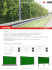### What is noise? - Personal Homepages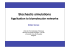### Mathematical Games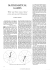### Schindler 3300 / Schindler 5300 Information on noise and vibration.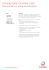### Bonus Appendix - Links to Product Sites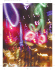### case study: channel 5 news - London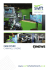### Population Dynamics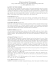### Jaramillo_AM_D_2013 - VTechWorks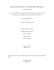### Noise Action Plan - Liverpool John Lennon Airport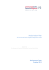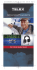### VorTech QuietDrive information packet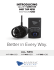### Low-Order Stochastic Mode Reduction for a Realistic Barotropic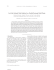### Operation and Service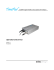### Model PC-100 Power Supply Control Module Operator`s Manual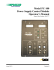### THREE DIMENSIONAL SYSTEMS Lecture 6: The Lorenz Equations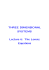### Analysis of Parametric Oscillatory Instability in Signal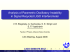### SUBSPACE IDENTIFICATION FOR LINEAR SYSTEMS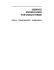### 023_Indiscriminate Use of Loudspeaker and its Legal Control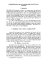### Nanotechnology Measurement Handbook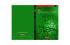### Coping with Incomplete Information